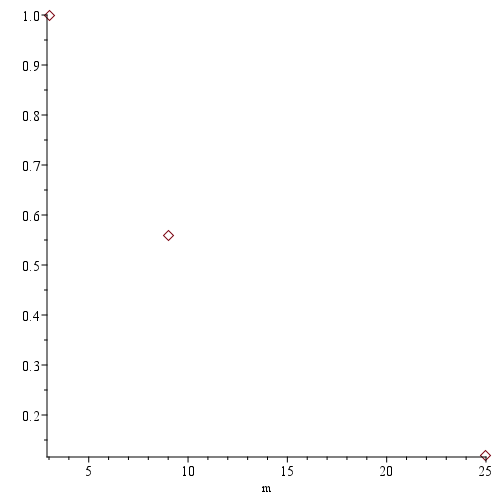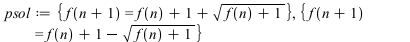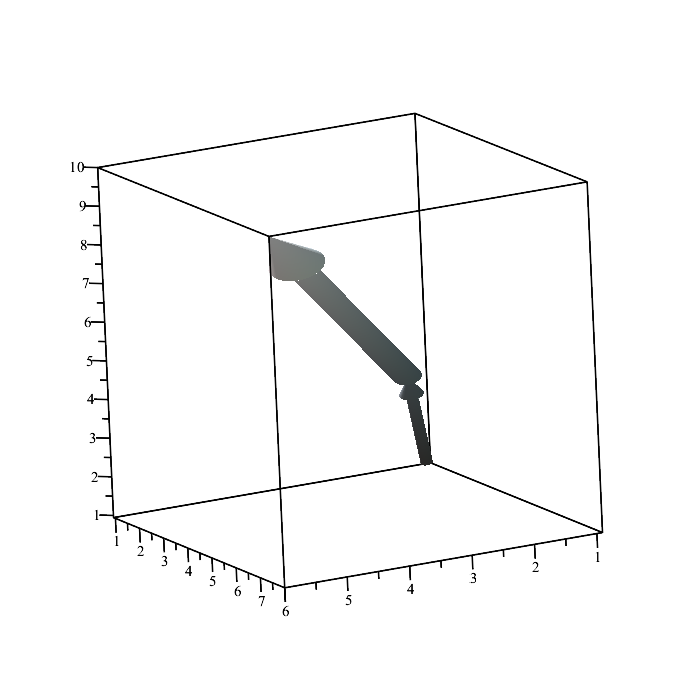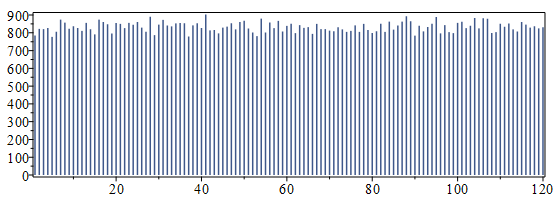Carl Love

## 26104 Reputation

11 years, 57 days
Himself
Wayland, Massachusetts, United States
My name was formerly Carl Devore.

## plot with useunits...

The regular plot command can be used for this. You just need to group the X and Y into a single argument:

plot(<X1 | Y>, style= point, symbolsize= 15, useunits= Unit~(['m', 1]));## Simple Maple code...

Your only have 1 equation (Eq 21), so it can only be solved for 1 variable at a time. Your stated "solutions" have solutions for 4 variables: omegaPsikappa__0, and h__1. If we take 3 of these as "given", we can easily solve for the 4th. In the worksheet below, I solve for omega.

 > restart:
 > Eq21:=     12*Psi^3*rho__3^2*D[1,1](w)(phi) +     (4*omega*rho__3^2 - 3*rho__2^2*Psi)*w(phi) +     Psi*rho__3^2*(rho__1 + 2*rho__3)*w(phi)^3 ;> w:= phi-> kappa__0 + kappa__1*(D(Xi)/Xi)(phi) + h__1*(Xi/D(Xi))(phi);> Xi:= phi-> (1+exp(phi))/exp(-2*phi);> Eq21a:= eval(  #Use given solutions for h__1, kappa__0, and Psi.     Eq21,     [         h__1= 0,         kappa__0= -5/2*kappa__1,         Psi= kappa__1/12*sqrt(-6*rho__1 - 12*rho__3)     ] );> solve({Eq21a}, {omega});>

## Extracting the odd part...

If 2 is the only base for which you want to do this, and you don't actually need the prime factorization of the remaining odd part, then there's a much faster way to do this. I mention this because factoring out the highest-possible power of 2 is a very common preliminary step in number-theory algorithms; so I'm guessing that that may be your goal. Here's how:

```OddPart:= (n::posint, x::algebraic)->
(k-> x^k*integerdivq2exp(n,k))(Bits:-FirstNonzeroBit(n, 'higher'))
:
#Random test case:
(p1,p2):= nextprime~(['rand(2^64..2^65)()'\$2])[];
p1, p2 := 24421176063119381659, 21314142271437361117
N:= 2^rand(9..99)()*p1*p2;
N := 266504407575115289384393504182133531188736

CodeTools:-Usage( ifactor(N) );
memory used=14.24MiB, alloc change=0 bytes,
cpu time=172.00ms, real time=175.00ms, gc time=0ns

9
(2)  (21314142271437361117) (24421176063119381659)

CodeTools:-Usage( OddPart(N,x) );
memory used=1.49KiB, alloc change=0 bytes,
cpu time=0ns, real time=0ns, gc time=0ns

9
520516421045147049578893562855729553103 x

```

## pde2 is not an equation, don't use lhs...

Your pde2 is not explicitly an equation because it doesn't consist of two expressions separated by =. Thus, it has neither a left side nor a right side. Thus, the command lhs (which stands for left-hand side) can't be used. This error is purely due to syntax, not math. If you intend pde2 to represent an expression implicitly equated to 0 (which is a very common and totally acceptable thing to do), then simply proceed with normal(pde2) instead of normal(lhs(pde2)), and your entire worksheet completes without error.

Regarding your tanh question: Did you intend that question to be in connection to the error? If so, the error has nothing to do with math. If you intended the tanh question to be independent of the error, I can attempt to answer it.

## simplify with side relations...

The simplify with side relations (see ?simplify,siderels) command can do it:

simplify(x^2+y^2, {x-y, y+a}, [x,y]);

## unprotect(_Z); `tools/genglobal`(_Z, 0, ...

The command to clear the counter on an automatically generated global variable such as _Z1 is `tools/genglobal`. Before each solve command, including the first, do

unprotect(_Z); `tools/genglobal`(_Z, 0, 'clear'):

However, it would be a better programming practice to "improve the program" in the manner @acer suggests. One reason that this is more robust is that if you and other people are developing the same code, they might not be aware that you've reset the counter.

`tools/genglobal` is undocumented. I figured out how to use it by reading its code via

showstat(`tools/genglobal`);

## p < 2%...

The following simulation, sampling over 2 million random drawings, shows that the probability that a random drawing would have no intra-family pairings is less than 2%:

```Fams:= [{\$1..3}, {\$4..7}, {\$8..11}, {\$12..15}, {16}]:
ValidDraw?:= (M::list(integer[1..16]))->
andmap(f-> f intersect {M[[f[]]][]} = {}, Fams)
:
V:= [
to 2^21 do
M:= combinat:-randperm(16);
if ValidDraw?(M) then M fi
od
]:
nops(V);
37276
evalf(%/2^21);
0.01777458191```

Using formal Statistics language, the p-value is less than 2%.

A well-known formula for combinatorial derangement says that the probability that no person is paired with themself is very close to exp(-1) ~ 36.8%. The exact value is  15549624751/42268262400, which equals exp(-1) to 14 decimal places.

## Allowed since Maple 2019...

Despite what @nm and @igor.v.proskurin said, what you want to do has been allowed since Maple 2019. It looks like you're using an older version, Maple 2018. Now, local declarations are allowed in a large number of positions within a procedure. I guess that that Programming Guide entry needs to be updated.

## D to differentiate procedures...

You should use D to define g, like this:

g:= D(f);

The problem with your g:= x-> diff(f(x), x) is that g(1) evaluates to diff(2, 1), which is nonsense because 1 isn't a variable.

## rsolve(..., makeproc)...

Your reqn has two solutions for f(n+1), and each of them specifies a different recurrence:

psol:= solve({f(n+1)=f(n)+sqrt(2*f(n+1)-f(n))}, {f(n+1)});I don't think that there's any hope of a symbolic solution for either of these (and the rsolves return unevaluated), but you may be able to make a numeric asymptotic analysis. In the following command, note that I've specified the initial condition as 15., with a decimal point, to force floating-point evaluations. If you don't do this, the expressions become extremely large with deeply nested square roots very fast.

F:= rsolve({psol, f(1)=15.}, f(n), makeproc);

Now you can explore, for example,

seq(F(k), k= 1..99);

If I used psol instead, the solution rapidly decays to 0.

## RootedVector, PlotVector...

Using package VectorCalculus, I'd do it with the commands RootedVector and PlotVector (definitely not PlotPositionVector). For example, we're given the following initial tail point, base, and vectors u and v:

base:= [1,1,1]:  u:= <1,2,3>:  v:= <4,5,6>:

We want to represent the vector sum u + v head-to-tail in that order:

VC:= VectorCalculus:
VC:-PlotVector([VC:-RootedVector(root= base, u), VC:-RootedVector(root= base+~u, v)]);There are numerous options to PlotVector to control the size, color, shape, etc., of the vectors; see its help page.

The same process works just as well for a 2D plot if all vectors and points have 2 components.

## Discrete uniform 1..120 using 3.7 expect...

From a Reply, I see that you really want to generate discrete uniform random numbers from 1..120 using a 6-sided die (note that dice is strictly plural, like mice and lice). The process can be simplified because 120 can be expressed as a product of positive integers all <= 6; specifically, 120 = 4*5*6. (Number theorists would say that 120 is {2, 3, 5}-smooth, the {2, 3, 5} being the primes <= 6.) Since those are three factors, it'll often be possible with three rolls of the 6-sided die. However, sometimes a roll will need to be discarded and rerolled. The expected number of rolls is exactly 3.7 (Maple-based proof below).

I use a multi-radix representation. This is similar to an alternate-base representation, but with a different base for each digit position. For any n in {\$120}, there exists a unique triple [d4, d5, d6] such that

n = 1 + 5*6*(d4 - 1) + 6*(d5 - 1) + (d6 - 1),   (formula 1)

where d4 in {\$4}, d5 in {\$5}, and d6 in {\$6}[*1] (proof outline in footnote). I developed this formula to make the arithmetic simple enough for your son. So, how do we generate d4 and d5 uniformly with a 6-sided die? For d4, roll the die, perhaps repeatedly, until it gives a number <= 4; for d5, do the analogous thing. The expected number of rolls for d_k (i.e., d4d5, etc.) is

sum(n*k/s*(1 - k/s)^(n-1), n= 1..infinity) assuming 0 < k, k <= s,

where s is the number of sides on the actual die, and n runs over all possible numbers of rolls. The sum simplifies to s/k, as you can check with Maple. (You can also look up the negative binomial distribution in Wikipedia.) So, in this particular case the expected number of rolls to get all three "digits" is 6/4 + 6/5 + 6/6 = 37/10 = 3.7

If n is not {2, 3, 5}-smooth, a slightly more-complicated process can be used, and the expected number of rolls will be slightly more.

Here's a Maple simulation for n = 120, which I coded to be easily modifiable to other numbers of sides of the die and appropriately smooth values of n

```restart
:
Roll_120:= module()
local
sides::And(posint, Not(1)):= 6,
ds:= rand(1..sides),
roll:= (k::And(posint, Not(1)))-> local r;
do Roll_Ct++; if (r:= ds()) <= k then return r fi od,
Coeffs:= (P-> P[-1]/~P)([seq[scan= `*`]](radices))  #[30, 6, 1]
;
export
ModuleApply:= ()-> (R-> R = 1 + inner(R-~1, Coeffs))(roll~(radices)),
Roll_Ct:= 0  #Count the rolls only to verify expectation.
;
end module
:
#Choose the hockey team!:
'Roll_120'() \$ 5;
[2, 5, 6] = 60, [2, 3, 4] = 46, [4, 5, 3] = 117, [1, 5, 2] = 26, [3, 2, 2] = 68
#If a card number were repeated, we'd simply reroll for that card.

Roll_120:-ExpectedRolls;
37 / 10

#Performance test for 100,000 triples:
Roll_120:-Roll_Ct:= 0:
Rolls:= CodeTools:-Usage([to 10^5 do Roll_120() od]):
memory used=74.07MiB, alloc change=-3.01MiB,
cpu time=766.00ms, real time=727.00ms, gc time=62.50ms

Roll_120:-Roll_Ct;  #Check expected roll count.
369526

#Check uniformity:
RRolls:= rhs~(Rolls):
[{Statistics:-Tally(RRolls)[]}[]];
[1 = 785, 2 = 822, 3 = 821, 4 = 827, 5 = 777, 6 = 806, 7 = 874, 8 = 857, 9 = 823, 10 = 837,
11 = 827, 12 = 811, 13 = 856, 14 = 820, 15 = 791, 16 = 874, 17 = 861, 18 = 848, 19 = 796, 20 = 855,
21 = 850, 22 = 826, 23 = 856, 24 = 845, 25 = 861, 26 = 829, 27 = 806, 28 = 890, 29 = 787, 30 = 846,
31 = 873, 32 = 841, 33 = 836, 34 = 853, 35 = 855, 36 = 854, 37 = 779, 38 = 842, 39 = 854, 40 = 827,
41 = 903, 42 = 814, 43 = 816, 44 = 797, 45 = 829, 46 = 835, 47 = 854, 48 = 819, 49 = 861, 50 = 868,
51 = 824, 52 = 802, 53 = 781, 54 = 880, 55 = 802, 56 = 858, 57 = 826, 58 = 867, 59 = 807, 60 = 839,
61 = 851, 62 = 798, 63 = 843, 64 = 828, 65 = 832, 66 = 794, 67 = 850, 68 = 821, 69 = 821, 70 = 812,
71 = 809, 72 = 831, 73 = 819, 74 = 805, 75 = 811, 76 = 842, 77 = 805, 78 = 850, 79 = 816, 80 = 799,
81 = 809, 82 = 851, 83 = 805, 84 = 863, 85 = 818, 86 = 841, 87 = 863, 88 = 893, 89 = 865, 90 = 784,
91 = 840, 92 = 808, 93 = 833, 94 = 851, 95 = 889, 96 = 797, 97 = 843, 98 = 804, 99 = 798, 100 = 856,
101 = 862, 102 = 826, 103 = 840, 104 = 883, 105 = 825, 106 = 882, 107 = 879, 108 = 799, 109 = 804, 110 = 851,
111 = 834, 112 = 852, 113 = 819, 114 = 807, 115 = 861, 116 = 846, 117 = 829, 118 = 837, 119 = 825, 120 = 831]

120, 100000

Statistics:-Histogram(RRolls, discrete, frequencyscale= absolute, size= 70*[8,3]);
```The process that you show in the Question has two major mathematical errors. (This is totally independent of the Maple problem of automatic simplification / premature evaluation that's already been addressed.):

1. A sum of independent nonconstant uniform random variables can never be uniform.
2. If r is a discrete uniform random variable on 1..n, then r mod d can only be uniform if d divides (or, quite trivially, if abs(d) > n).

Footnote:

[*1] Outline of a proof of the existence and uniqueness of the multi-radix representation

n = 1 + 5*6*(d4 - 1) + 6*(d5 - 1) + (d6 - 1).

Let d6:= irem(n-1, 6, 'q') + 1d5:= irem(q, 5, 'q') + 1d4:= irem(q, 4) + 1. Each of these steps is defined and produces a unique value.

## It works...

The Submit Software Change Request feature of MaplePrimes works. You may have simply experienced some temporary server problem or Internet problem.

## Mental solution; no Maple...

My mental solution without Maple:

rel(3) is obviously true; rel(2) can be verified by elementary arithmetic. For n > 3, divide both sides by ((n-3)*2)^(1/(n-1)). The equation becomes (n-3)^1 * 2^1 = (n-1)*2^1 - 4, which reduces to 2*n-6 = 2*n-6.

## Close-to-original workaround...

Here's a workaround that's as close to the "spirit" of the original command as I think is possible. It uses subs to perform simultaneous substitutions by making the first argument to subs a list.

subs(op(expr)=~ [a,b,c,d], expr)

(As always, my posting of a "workaround" is unrelated to whether I think there's a bug. In this case, I do think that there's a bug.)

 1 2 3 4 5 6 7Last Page 1 of 378
﻿